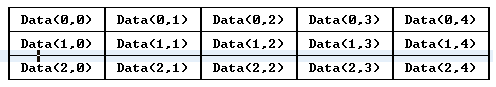﻿ Multidimensional Arrays
 Arrays can have more than one dimension. A multidimensional array has multiple subscripts to select an individual element.   A 2-dimensional array can be visualized as a grid of rows and columns that contain data. For example, a 3-by-5 array named "Data" would look like this:Notice that the order of the subscripts is row followed by column. The rows increase going down, and the columns increase going to the right. (You can reverse this order and think of a 3-by-5 array as having 3 columns and 5 rows, but this is not the order used by matrices and constant arrays.) This kind of data structure is used for many things, such as board games, matrix calculations, and pixel coordinates.   The 2-dimensional array shown above can be set up and used as follows:           integer Data(3,5), I, J;         begin         for I:= 0, 3-1 do             for J:= 0, 5-1 do                 Data(I,J):= 0;         Data(1,3):= 42;         . . .   More dimensions can be easily added. Here is a 3-by-5-by-8 array, this time using a real variable:           real    Data(3,5,8);         int     I, J, K;         begin         for I:= 0, 3-1 do             for J:= 0, 5-1 do                 for K:= 0, 8-1 do                     Data(I,J,K):= 0.0;         Data(1,3,7):= 42.0;         . . .   Character arrays can also be multidimensional. For example:           character String(100,80);   This reserves space for 100 strings that are each 80 bytes long. Note that the number of bytes is specified by the last dimension. Single bytes are accessed using a subscript:           String(I,J):= ^A;         ChOut(0, String(99,3));